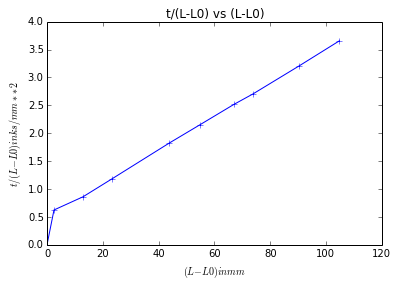# Chapter 10:Mass Transfer¶

## Example no:10.1,Page no:580¶

In :
#Variable declaration
x=1e-3  #Thickness of stagnant air film
D=1.8e-5 #Difffusivity of ammonia
R=8314  #Gas constant
T=295  #Temperature
P=101.3e3  #Total Pressure

#Calculation
import math
#If the subscripts 1 and 2 refer to the two sides of the stagnant layer and
#the subscripts A and B refer to ammonia and air respectively,
P_A1=.50*P
P_A2=0
P_B1=P-P_A1
P_B2=P-P_A2
P_BM=(P-P_A1)/math.log(P/P_A1)
#Thus, substituting in equation 10.31 gives:
N_A=(-D/(R*T*x))*(P/P_BM)*(P_A2-P_A1)

#Result
print"The rate of diffusion of ammonia through the layer = %.2e"%N_A,"kmol/m**2*s"

The rate of diffusion of ammonia through the layer = 5.15e-04 kmol/m**2*s


## Example no:10.2,Page no:582¶

In :
#Variable declaration
import numpy as np
%matplotlib inline
th=np.array([0,0,3,7,22,32,46,55,80,106])       #Time in hours
tm=np.array([0,26,5,36,16,38,50,25,22,25])      #Time in min
tim=*10
#Conversion to kilo seconds
for i in range(0,10):
tm[i]=tm[i]*60.0
th[i]=th[i]*3600.0
tim[i]=(tm[i]+th[i])/1000.0

L=np.array([0,2.5,12.9,23.2,43.9,54.7,67.0,73.8,90.3,104.8])    #in mm
Lo=L

#Calculation
x=L-Lo
y=*10
for j in range(1,10):
y[j]=tim[j]/(L[j]-Lo)
plot(x,y,marker='+')
plt.title('t/(L-L0) vs (L-L0)')
plt.xlabel('$(L-L0) in mm$')
plt.ylabel('$t/(L-L0) in ks/mm**2$')

#Calculation of slope
s=(y-y)/(x-x)*10**3*10**6
Vl=22.4                  #Molar volume in litres
den=1540                 #Density in kg/m**3
T0=273
T=321
vp=37.6                  #vapour pressure in kPa
P0=101.3                 #PRessue in kPa
M=154
Ct=T0/(Vl*T)
Ca=(vp*Ct)/P0
Cb1=Ct
Cb2=(P0-vp)*Ct/P0
Cbm=(Cb1-Cb2)/math.log(Cb1/Cb2)
#Diffusivity calculation
D=den*Cbm/(2*M*Ca*Ct*s)

#Result
show()
print"\nSlope is %.1e"%s,"s/m**2"
print"\nDiffusivity is %.2e"%D," m**2/s"

Populating the interactive namespace from numpy and matplotlibSlope is 3.1e+07 s/m**2

Diffusivity is 9.17e-06  m**2/s


## Example no:10.3,Page no:585¶

In :
#Variable declaration
P=101.3e3  #pressure of the operating column
T=295.0  #Temperature of the operating column
P_A=7.0e3  #partial pressure of ammonia
x=1.0e-3  #=(y1-y2)Thickness of stationary gas film
D=2.36e-5  #Diffusivity of ammonia

#Calculation
import math
C_A=(1.0/22.4)*(273.0/T)*(P_A/P) #=(C_A1-C_A2)Concentration of ammonia gas
#X=C_T/C_BM
X=P*math.log(P/(P-P_A))/(P-(P-P_A))
#From equation 10.33
N_A_=(D/x)*X*(C_A)

#Result
print"The transfer rate per unit area = %.2e"%N_A_,"kmol/m**2*s"

The transfer rate per unit area = 6.98e-05 kmol/m**2*s


## Example no:10.4,Page no:606¶

In :
#Variable declaration
Q=3e-6  #Flow rate of water
Meu=1e-3  #Viscosity of water
D=1.5e-9  #diffusivity of carbon dioxide in water
rho=1e3  #Density of water

#Calculation
s=(Q*1e2*Meu*3/(rho*9.81))**(1.0/3.0) #Thickness of film
A=0.95
y=s*(1-A)**0.5 #The distance below the free surface
t=(1.305/1.822)**2
Us=rho*9.81*s**2/(2*Meu) #surface velocity
L=Us*t #The maximum lend=gth of column

#Result
print"\n The maximum length of column =",round(L,2)," m"

 The maximum length of column = 0.51  m


## Example no:10.5,Page no:608¶

In :
#Variable declaration
N_dot=50  #Initial maas transfer rate
D=1.8e-9  #Diffusivity of gas in liquid phase
C_bg=(1/22.4)*(273.0/293.0)  #bulk gas concentration

#Calculation
import math
N_C=N_dot*C_bg  #Initial maas transfer rate in terms of cocentration
h=N_C/0.04 # Effective Mass transfer coefficient
R=1/h #Equivalent resistance
R_l=R*9  #Liquid phase resistance
h_l=1/R_l  #Liquid phase coefficient
#From equation 10.113 and using liquid phase resistance
t=R_l**2/(math.pi/D)

#Result
print"The required time is =%.2e"%t,"s"

The required time is =1.72e-11 s


## Example no:10.6,Page no:608¶

In :
#Variable declaration
import math
#Diffusivity of CO2 in ethanol
D=4*10**-9                #in m**2/sw
t=100                 #Time in sec
import sympy
Cai=sympy.Symbol('x')

#Calculation
y=[0,10**-3]

mole=*3
for i in range(0,2):
mole[i]=((2*math.sqrt(D*t/math.pi)*math.exp(-y[i]**2/(4*D*t)))-(y[i]*math.erfc(y[i]/(2*math.sqrt(D*t)))))

ret=(mole-mole)/mole

#Result
print"Proportion retained is %d"%(ret*100),"%"

Proportion retained is 83 %


## Example no:10.8,Page no:621¶

In :
#Variable declaration
L=825.0e-3  #length of the tube
d=15.0e-3  #diameter of the tube
P_i=7.5e3  #Partial pressure of ammonia at inlet
P_o=2.0e3  #Partial pressure of ammonia at inlet
A_r=2.0e-5  #Air rate
P=101.3e3  #Atmospheric pressure

#Calculation
import math
D_F_m=(P_i-P_o)/math.log(P_i/P_o) #Mean driving force
A_absorbd=A_r*((P_i/(P-P_i))-(P_o/(P-P_o)))
A_w=math.pi*d*L #Wetted surface
K_G=(A_absorbd/(A_w*D_F_m)) #Overall transfer coefficient

#Result
print"Overall Transfer coefficient =%.2e"%K_G,"kmol/[m**2 s (N/m**2)]"

Overall Transfer coefficient =7.40e-09 kmol/[m**2 s (N/m**2)]


## Example no:10.9,Page no:628¶

In :
#Variable declaration

z=0.693
#Calculation
import math
ratio=(math.exp(math.sqrt(z))+math.exp(-math.sqrt(z))-4.0)/(2.0*(1-math.exp(-math.sqrt(z))-math.exp(math.sqrt(z))))

#Result
print"The final ratio is",round(ratio,1)

The final ratio is 0.4


## Example no:10.10,Page no:629¶

In :
#Variable declaration
#After denoting the original conditions by subscript 1,
#The mass transfer rate (moles/unit area and unit time) is given by
#N_A2=0.83*N_A1

#Calculation
import math
n=2*(math.log(0.83/(1.35)**0.5)/math.log(0.8))-1

#Result
print"n =",round(n,2)
print"Thus the reaction is of second order"

n = 2.01
Thus the reaction is of second order


## Example no:10.11,Page no:630¶

In :
#Variable declaration
k = 2.5*10**-6            #[s**-1] Rate constant
E = 2.643*10**7           #[J/kmol] Energy of Activation
R = 8314                 #[J/kmol.K] Universal gas contss
D = 10**-9                #[m**2/s] MOlecular diffuisivity
L = .01                  #[m] Film Thickness

#Calculation
import math
#At T =293K
T = 293                 #[K] temperature
A = k/math.exp(-E/(R*T))     #[s**-1]
e = math.exp(-2*L*math.sqrt(k/D))
N = math.sqrt(k/D)*(1+e)/(1-e)  #Consider relative Solubility at 293 K be unity
#At T =313K
T2 = 313                 #[K] temperature
k2 = A*math.exp(-E/(R*T2))     #[s**-1]
e2 = math.exp(-2*L*math.sqrt(k2/D))
N2 = .8*math.sqrt(k2/D)*(1+e2)/(1-e2)  #Consider relative Solubility at 313 K be .8 wrt that of 293K

Nr = N2/N

#Result
print'Change in mass transfer rate is given by factor',round(Nr,2)

Change in mass transfer rate is given by factor 0.86


## Example no:10.12,Page no:643¶

In :
#Variable declaration
k=5e-4 #first order rate constant
D_e=2e-9 #effective diffusivity of reactants in the pores of the particles

#Calculation
import math
lamda=(k/D_e)**0.5
# (i) For the platelet of thickness 8 mm,
L=0.5*(8e-3)
phi=lamda*L #thiele modulus
#From equation 10.202, the effectiveness factor 'eta' is given by:
eta=(1/phi)*math.tanh(phi)
#(ii) For the sphere of diameter 10 mm, r_o = 0.005 m**-1.
r_o=5e-3
phi_o=lamda*r_o #Thiele modulus
#From equation 10.212, the effectiveness factor 'eta' is given by:
eta_o=(3/phi_o)*((1.0/math.tanh(phi_o))-(1/phi_o))

#result
print"\n (i) Thiele modulus =",phi
print"\n     The effectiveness factor =",round(eta,3)
print"\n (ii) Thiele modulus =",phi_o
print"\n     The effectiveness factor =",round(eta_o,3)

 (i) Thiele modulus = 2.0

The effectiveness factor = 0.482

(ii) Thiele modulus = 2.5

The effectiveness factor = 0.736


## Example no:10.13,Page no:644¶

In :
#Variable declaration
D_e=1e-5 #Effective diffusivity for the reactants in the catalyst particle
k=14.4 #first order rate constant
L=2.5e-3

#Calculation
import math
lamda=(k/D_e)**0.5
phi=(k/D_e)**0.5*(L) #Thiele modulus
#From equation 10.202, the effectiveness factor,
eta=(1/phi)*math.tanh(phi)
#The concentration profile is given by equation 10.198
y=1.25e-3
C_Ai=0.15
C_A=C_Ai*(math.cosh(lamda*y)/math.cosh(lamda*L))

#Result
print"\n (i) The effectiveness factor =",round(eta,3)
print"\n (ii) The concentration of reactant at a position half-way between the centre and the outside of the\n\t pellet =",round(C_A,3),"kmol/m**3"

 (i) The effectiveness factor = 0.332

(ii) The concentration of reactant at a position half-way between the centre and the outside of the
pellet = 0.035 kmol/m**3


## Example no:10.14,Page no:645¶

In :
#Variable declaration
R_r=8.2e-2 #reaction rate when concentration =0.011 kmol/m**3
D_e=7.5e-8 #Effective diffusivity

#Calculation
#catalyst = eta*k*C_Ai (equation 10.217),
#eta=phi_L**-1
#Substituting numerical values in prev eqn:
k=(1.217*R_r/0.011)**2
phi_L=1.217*(k)**0.5
eta=phi_L**-1

#Result
print"Effectiveness factor =",round(eta,4)

Effectiveness factor = 0.0906